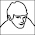## 18 Apr 2011

### Test Difference Between Diversity-Indices of Two Samples with Abundance Data

I adapted a permutation test from the PAST Software (Hammer & Harper, http://folk.uio.no/ohammer/past/diversity.html) that tests difference between diversity-indices of two samples with abundance data in R... See the example below:

```### two samples with abundance data:
require(vegan)

### two samples with abundance data:
### 1st sample of counts (numbers representing species 1-15)
dat1 <- c(1,2,0,3,0,1,4,20,0,2,21,3,15,23,30)

### 2nd sample of counts (numbers representing species 1-15)
dat2 <- c(0,1,0,1,0,0,1,10,0,0,29,1,22,30,25)

(div1=diversity(t(dat1),"shannon"))
(div2=diversity(t(dat2),"shannon"))
(rich1=sum(dat1>0))
(rich2=sum(dat2>0))

(tr.diff.div=abs(div1-div2))          ### observed difference
(tr.diff.rich=abs(rich1-rich2))       ### ...

K=2000

pop.diff.div <- pop.diff.rich <- rep(NA,K)      ### dataframe for null population of differences
pop.diff.div=tr.diff.div
pop.diff.rich=tr.diff.rich

for(i in 2:K){                        ### loop to generate null pop.diff. of differences

ind1<-sum(dat1)                     ### sum of individuals sample no.1
ind2<-sum(dat2)                     ### sum of ... no.2
pool<-c(rep(1:length(dat1),dat1),   ### pooled sample with numbers representing species
rep(1:length(dat2),dat2))   ### replicated as often as no. of individuals

temp1=sample(pool,ind1,replace=T)   ### resample no.1
temp2=sample(pool,ind2,replace=T)   ### resample no.2

### calculate diversity:
div1.temp=diversity(t(tabulate(temp1)),"shannon")
div2.temp=diversity(t(tabulate(temp2)),"shannon")

rich1.temp=sum(tabulate(temp1)>0)
rich2.temp=sum(tabulate(temp2)>0)

pop.diff.div[i]=abs(div1.temp-div2.temp)
pop.diff.rich[i]=abs(rich1.temp-rich2.temp)
}

(p.div=sum(pop.diff.div>=abs(tr.diff.div))/K)
(p.rich=sum(pop.diff.rich>=abs(tr.diff.rich))/K)

### diagramms to show null-distributions with obs. differences
par(mfrow=c(2,1))
hist(pop.diff.div)
abline(v=tr.diff.div,lty=3,col=2,lwd=2)

### diagramms to show null-distributions with obs. differences
hist(pop.diff.rich)
abline(v=tr.diff.rich,lty=3,col=2,lwd=2)
```

Taking into account what Jari commented below (simplifying code & fixing row and column totals - still having in mind that the test may be anti-conservative!)
```dat1 <- c(1,2,0,3,0,1,4,20,0,2,21,3,15,23,30)
dat2 <- c(0,1,0,1,0,0,1,10,0,0,29,1,22,30,25)

(div1=diversity(t(dat1)))
(div2=diversity(t(dat2)))

dat <- rbind(dat1, dat2)

B = 2000

sim <- r2dtable(B - 1, rowSums(dat), colSums(dat))
div <- sapply(sim, diversity)
pop.diff.div <- abs(div[1, ] - div[2, ])

(p <- sum(pop.diff.div >= abs(div1 - div2)) / B)
hist(pop.diff.div); abline(v = abs(div1 - div2))
```
Now with null model and vegan::oecosimu
```### two samples with abundance data:
### 1st sample of counts (numbers representing species 1-15)
dat1 <- c(1,2,0,3,0,1,4,20,0,2,21,3,15,23,30)

### 2nd sample of counts (numbers representing species 1-15)
dat2 <- c(0,1,0,1,0,0,1,10,0,0,29,1,22,30,25)

dat <- rbind(dat1, dat2)

divdiff <- function(x) abs(diversity(x[1, ]) - diversity(x[2, ]))
oecosimu(dat, divdiff, "r2dtable", nsimul = 1999)```

1.Thanks! How would you modify this for comparing more than two groups?

1...the most simple way is to do each comparison and apply a correction for multiple comparisons (Bonferroni, i.e.)i

2.The test defines a community null model with constraints: (i) row sums (plot totals) are fixed, and (ii) species sampling probabilities are proportional to column sums (species totals). In this way column sums are close observed values but not fixed to column totals. It would be possible to have exact species totals (instead of approximate totals) using standard R function r2dtable. This allows faster and simpler code:

dat <- rbind(dat1, dat2)
sim <- r2dtable(1999, rowSums(dat), colSums(dat))
div <- sapply(sim, diversity)
pop.diff.div <- abs(div[1,]-div[2,])

Where pop.diff.div is the same as in the original script (but with modified null model)

Quantitative null models are a dangerous and poorly known territory, and I would warn against using this simple models except after thorough testing. The major problem is that when individuals are redistributed freely, the variance of generated communities is usually much too low, and tests are too often "significant". Careful testing is the minimum requirement.

The development version of vegan at http://www.r-forge.r-project.org/ has a new infrastructure for community null models. This version also supports several quantitative null models, though not the one in the original message. However it would be easy to implment (in vegan naming scheme that would become "r1_ind"), and the new version also allows adding user-defined null models. The above test can be analysed in vegan::oecosimu quite easily.

Cheers, Jari Oksanen

1.Thanks - this helped a lot! I'll try to implement your suggestions (having in mind that the tests may be anti-conservative..).